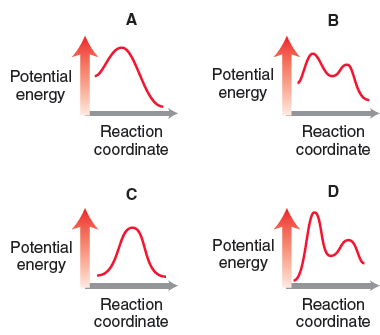Problem: Consider the following four energy diagrams: Which diagrams correspond with a one-step mechanism?

FREE Expert Solution
83% (236 ratings)
Problem Details

Consider the following four energy diagrams:

Which diagrams correspond with a one-step mechanism?What scientific concept do you need to know in order to solve this problem?

Our tutors have indicated that to solve this problem you will need to apply the Energy Diagram concept. You can view video lessons to learn Energy Diagram Or if you need more Energy Diagram practice, you can also practice Energy Diagram practice problems .

What is the difficulty of this problem?

Our tutors rated the difficulty of Consider the following four energy diagrams: Which diagrams... as low difficulty.

How long does this problem take to solve?

Our expert Organic tutor, Chris took 1 minute to solve this problem. You can follow their steps in the video explanation above.

What professor is this problem relevant for?

Based on our data, we think this problem is relevant for Professor Braunschweig & Harding's class at HUNTER.

What textbook is this problem found in?

Our data indicates that this problem or a close variation was asked in . You can also practice practice problems .